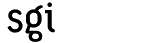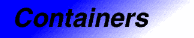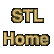# bitset<N>Category: containers Component type: type

### Description

Bitset is very similar to vector<bool> (also known as bit_vector): it contains a collection of bits, and provides constant-time access to each bit. There are two main differences between bitset and vector<bool>. First, the size of a bitset cannot be changed: bitset's template parameter N, which specifies the number of bits in the bitset, must be an integer constant. Second, bitset is not a Sequence; in fact, it is not an STL Container at all. It does not have iterators, for example, or begin() and end() member functions. Instead, bitset's interface resembles that of unsigned integers. It defines bitwise arithmetic operators such as &=, |=, and ^=.

In general, bit 0 is the least significant bit and bit N-1 is the most significant bit.

### Example

```int main() {

bitset<12> x;

cout << "Enter a 12-bit bitset in binary: " << flush;
if (cin >> x) {
cout << "x =        " << x << endl;
cout << "As ulong:  " << x.to_ulong() << endl;
cout << "And with mask: " << (x & mask) << endl;
cout << "Or with mask:  " << (x | mask) << endl;
}
}
```

### Definition

Defined in the standard header bitset.

### Template parameters

Parameter Description Default
N A nonzero constant of type size_t: the number of bits that the bitset contains.

### Model of

Assignable, Default Constructible, Equality Comparable

### Type requirements

N is a constant integer expression of a type convertible to size_t, and N is a positive number.

None.

### Members

Member Where defined Description
reference bitset A proxy class that acts as a reference to a single bit.
bitset() Default Constructible The default constructor. All bits are initially zero.
bitset(unsigned long val) bitset Conversion from unsigned long.
bitset(const bitset&) Assignable Copy constructor.
bitset& operator=(const bitset&) Assignable Assignment operator.
```template<class Char, class Traits, class Alloc>
explicit
bitset(const basic_string<Char,Traits,Alloc>& s,
size_t pos = 0,
size_t n =
basic_string<Char,Traits,Alloc>::npos)
```
bitset Conversion from string.
bitset& operator&=(const bitset&) bitset Bitwise and.
bitset& operator|=(const bitset&) bitset Bitwise inclusive or.
bitset& operator^=(const bitset&) bitset Bitwise exclusive or.
bitset& operator<<=(size_t) bitset Left shift.
bitset& operator>>=(size_t) bitset Right shift.
bitset operator<<(size_t n) const bitset Returns a copy of *this shifted left by n bits.
bitset operator>>(size_t n) const bitset Returns a copy of *this shifted right by n bits.
bitset& set() bitset Sets every bit.
bitset& flip() bitset Flips the value of every bit.
bitset operator~() const bitset Returns a copy of *this with all of its bits flipped.
bitset& reset() bitset Clears every bit.
bitset& set(size_t n, int val = 1) bitset Sets bit n if val is nonzero, and clears bit n if val is zero.
bitset& reset(size_t n) bitset Clears bit n.
bitset flip(size_t n) bitset Flips bit n.
size_t size() const bitset Returns N.
size_t count() const bitset Returns the number of bits that are set.
bool any() const bitset Returns true if any bits are set.
bool none() const bitset Returns true if no bits are set.
bool test(size_t n) const bitset Returns true if bit n is set.
reference operator[](size_t n) bitset Returns a reference to bit n.
bool operator[](size_t n) const bitset Returns true if bit n is set.
unsigned long to_ulong() const bitset Returns an unsigned long corresponding to the bits in *this.
```template<class Char, class Traits, class Alloc>
basic_string<Char,Traits,Alloc> to_string() const
```
bitset Returns a string representation of *this.
bool operator==(const bitset&) const Equality Comparable The equality operator.
bool operator!=(const bitset&) const Equality Comparable The inequality operator.
bitset operator&(const bitset&, const bitset&) bitset Bitwise and of two bitsets. This is a global function, not a member function.
bitset operator|(const bitset&, const bitset&) bitset Bitwise or of two bitsets. This is a global function, not a member function.
bitset operator^(const bitset&, const bitset&) bitset Bitwise exclusive or of two bitsets. This is a global function, not a member function.
```template <class Char, class Traits,
size_t N>
basic_istream<Char,Traits>&
operator>>(basic_istream<Char,Traits>&,
bitset<N>&)
```
bitset Extract a bitset from an input stream.
```template <class Char, class Traits,
size_t N>
basic_ostream<Char,Traits>&
operator>>(basic_ostream<Char,Traits>&,
const bitset<N>&)
```
bitset Output a bitset to an output stream.

### New members

These members are not defined in the Assignable, Default Constructible, or Equality Comparable requirements, but are specific to bitset.
Member Description
reference A proxy class that acts as a reference to a single bit. It contains an assignment operator, a conversion to bool, an operator~, and a member function flip. It exists only as a helper class for bitset's operator[]. That is, it supports the expressions x = b[i], b[i] = x, b[i] = b[j], x = ~b[i], and b[i].flip(). (Where b is a bitset and x is a bool.)
bitset(unsigned long val) Conversion from unsigned long. Constructs a bitset, initializing the first min(N, sizeof(unsigned long) * CHAR_BIT) bits to the corresponding bits in val and all other bits, if any, to zero.
```template<class Char, class Traits, class Alloc>
explicit
bitset(const basic_string<Char,Traits,Alloc>& s,
size_t pos = 0,
size_t n =
basic_string<Char,Traits,Alloc>::npos)
```
Conversion from string. Constructs a bitset, initializing the first M bits to the corresponding characters in s, where M is defined as min(N, min(s.size() - pos, n)). Note that the highest character position in s, not the lowest, corresponds to the least significant bit. That is, character position pos + M - 1 - i corresponds to bit i. So, for example, bitset(string("1101")) is the same as bitset(13ul). This function throws out_of_range if pos > s.size(), and invalid_argument if any of the characters used to initialize the bits are anything other than 0 or 1.
bitset& operator&=(const bitset&) Bitwise and.
bitset& operator|=(const bitset&) Bitwise inclusive or.
bitset& operator^=(const bitset&) Bitwise exclusive or.
bitset& operator<<=(size_t n) Left shift, where bit 0 is considered the least significant bit. Bit i takes on the previous value of bit i - n, or zero if no such bit exists.
bitset& operator>>=(size_t n) Right shift, where bit 0 is considered the least significant bit. Bit i takes on the previous value of bit i + n, or zero if no such bit exists.
bitset operator<<(size_t n) const Returns a copy of *this shifted left by n bits. Note that the expression b << n is equivalent to constructing a temporary copy of b and then using operator<<=.
bitset operator>>(size_t n) const Returns a copy of *this shifted right by n bits. Note that the expression b >> n is equivalent to constructing a temporary copy of b and then using operator>>=.
bitset& set() Sets every bit.
bitset& flip() Flips the value of every bit.
bitset operator~() const Returns a copy of *this with all of its bits flipped.
bitset& reset() Clears every bit.
bitset& set(size_t n, int val = 1) Sets bit n if val is nonzero, and clears bit n if val is zero. Throws out_of_range if n >= N.
bitset& reset(size_t n) Clears bit n. Throws out_of_range if n >= N.
bitset flip(size_t n) Flips bit n. Throws out_of_range if n >= N.
size_t size() const Returns N.
size_t count() const Returns the number of bits that are set.
bool any() const Returns true if any bits are set.
bool none() const Returns true if no bits are set.
bool test(size_t n) const Returns true if bit n is set. Throws out_of_range if n >= N.
reference operator[](size_t n) Returns a reference to bit n. Note that reference is a proxy class with an assignment operator and a conversion to bool, which allows you to use operator[] for assignment. That is, you can write both x = b[n] and b[n] = x.
bool operator[](size_t n) const Returns true if bit n is set.
unsigned long to_ulong() const Returns an unsigned long corresponding to the bits in *this. Throws overflow_error if it is impossible to represent *this as an unsigned long. (That is, if N is larger than the number of bits in an unsigned long and if any of the high-order bits are set.
```template<class Char, class Traits, class Alloc>
basic_string<Char,Traits,Alloc> to_string() const
```
Returns a string representation of *this: each character is 1 if the corresponding bit is set, and 0 if it is not. In general, character position i corresponds to bit position N - 1 - i. Note that this member function relies on two language features, member templates and explicit function template argument specification, that are not yet universally available; this member function is disabled for compilers that do not support those features. Note also that the syntax for calling this member function is somewhat cumbersome. To convert a bitset b to an ordinary string, you must write
```b.template to_string<char, char_traits<char>, allocator<char> >()
```
bitset operator&(const bitset&, const bitset&) Bitwise and of two bitsets. This is a global function, not a member function. Note that the expression b1 & b2 is equivalent to creating a temporary copy of b1, using operator&=, and returning the temporary copy.
bitset operator|(const bitset&, const bitset&) Bitwise or of two bitsets. This is a global function, not a member function. Note that the expression b1 | b2 is equivalent to creating a temporary copy of b1, using operator|=, and returning the temporary copy.
bitset operator^(const bitset&, const bitset&) Bitwise exclusive or of two bitsets. This is a global function, not a member function. Note that the expression b1 ^ b2 is equivalent to creating a temporary copy of b1, using operator^=, and returning the temporary copy.
```template <class Char, class Traits,
size_t N>
basic_istream<Char, Traits>&
operator>>(basic_istream<Char,Traits>& is,
bitset<N>& x)
```
Extract a bitset from an input stream. This function first skips whitespace, then extracts up to N characters from the input stream. It stops either when it has successfully extracted N character, or when extraction fails, or when it sees a character that is something other than 1 (in which case it does not extract that character). It then assigns a value to the bitset in the same way as if it were initializing the bitset from a string. So, for example, if the input stream contains the characters "1100abc", it will assign the value 12ul to the bitset, and the next character read from the input stream will be a.
```template <class Char, class Traits,
size_t N>
basic_ostream<Char,Traits>&
operator>>(basic_ostream<Char,Traits>& os,
const bitset<N>& x)
```
Output a bitset to an output stream. This function behaves as if it converts the bitset to a string and then writes that string to the output stream. That is, it is equivalent to
```os << x.template to_string<Char,Traits,allocator<Char> >()
```

### Notes